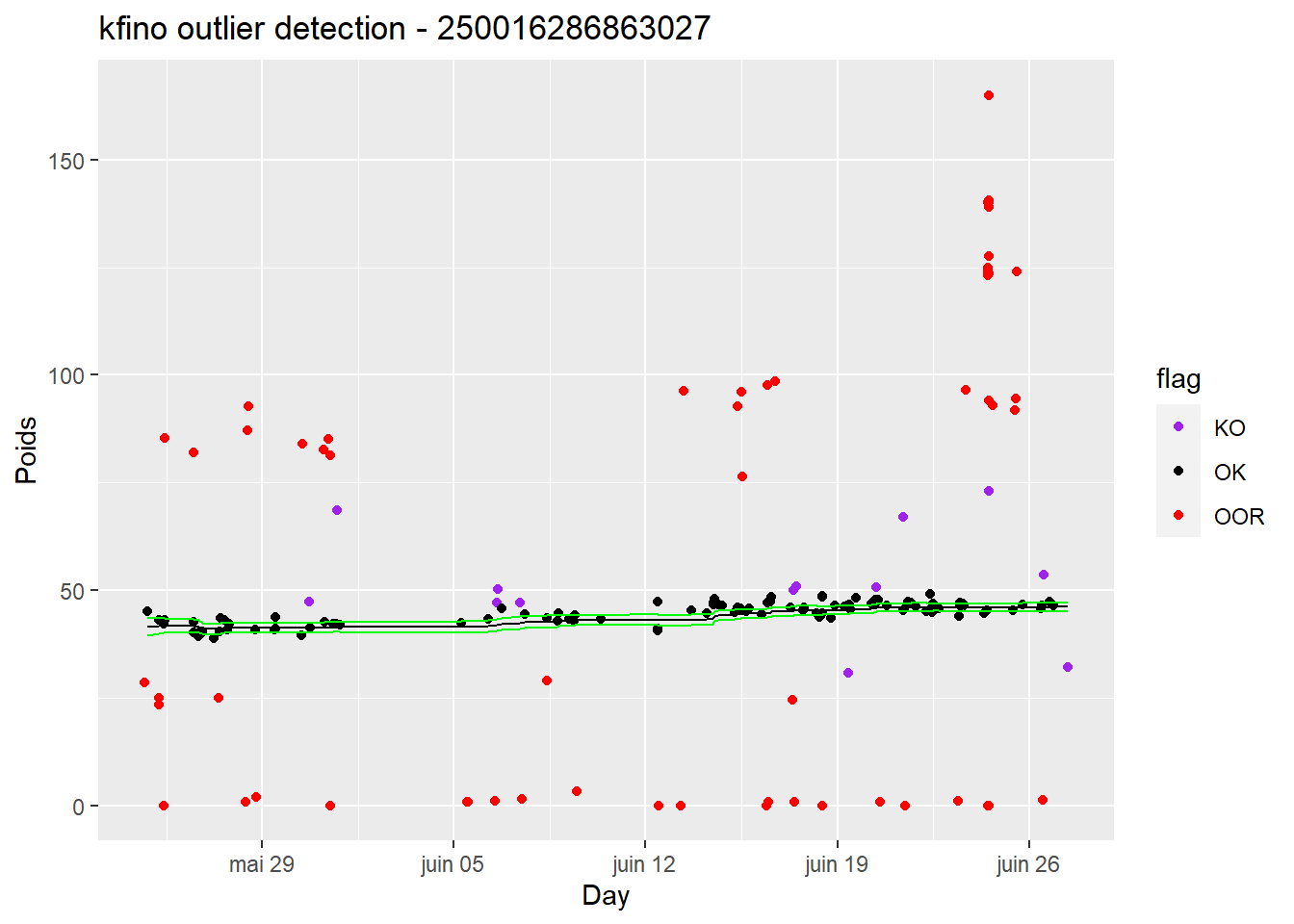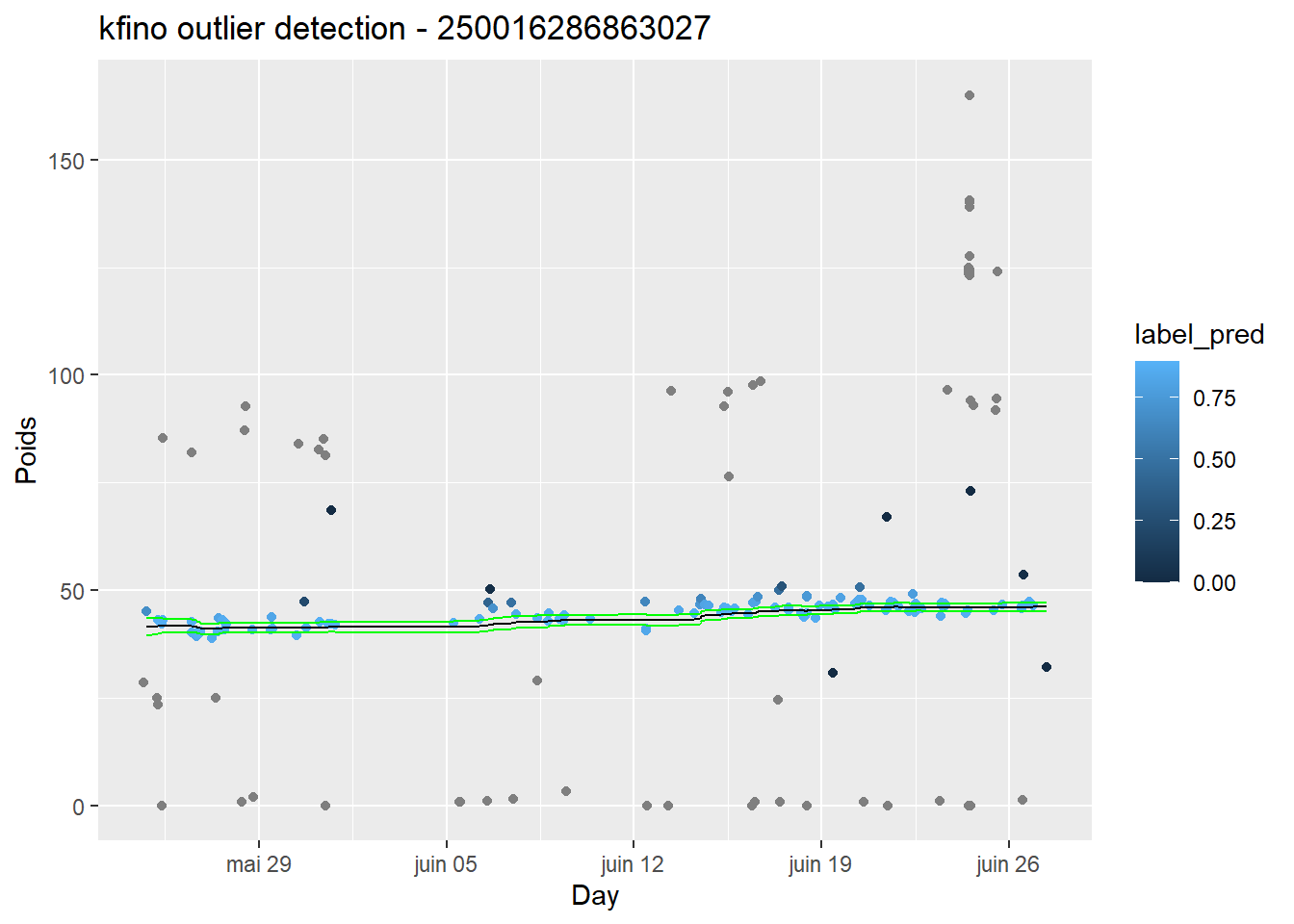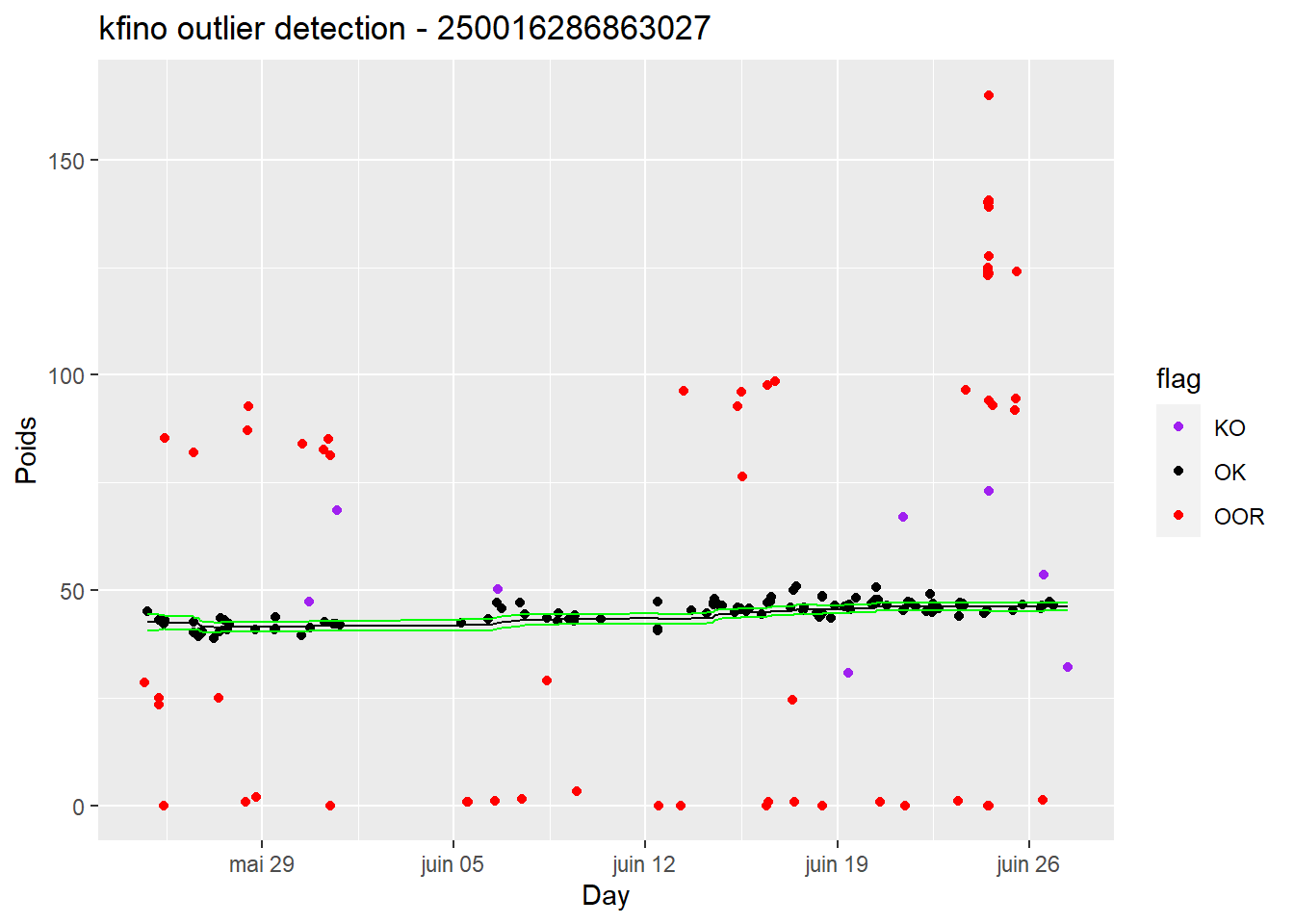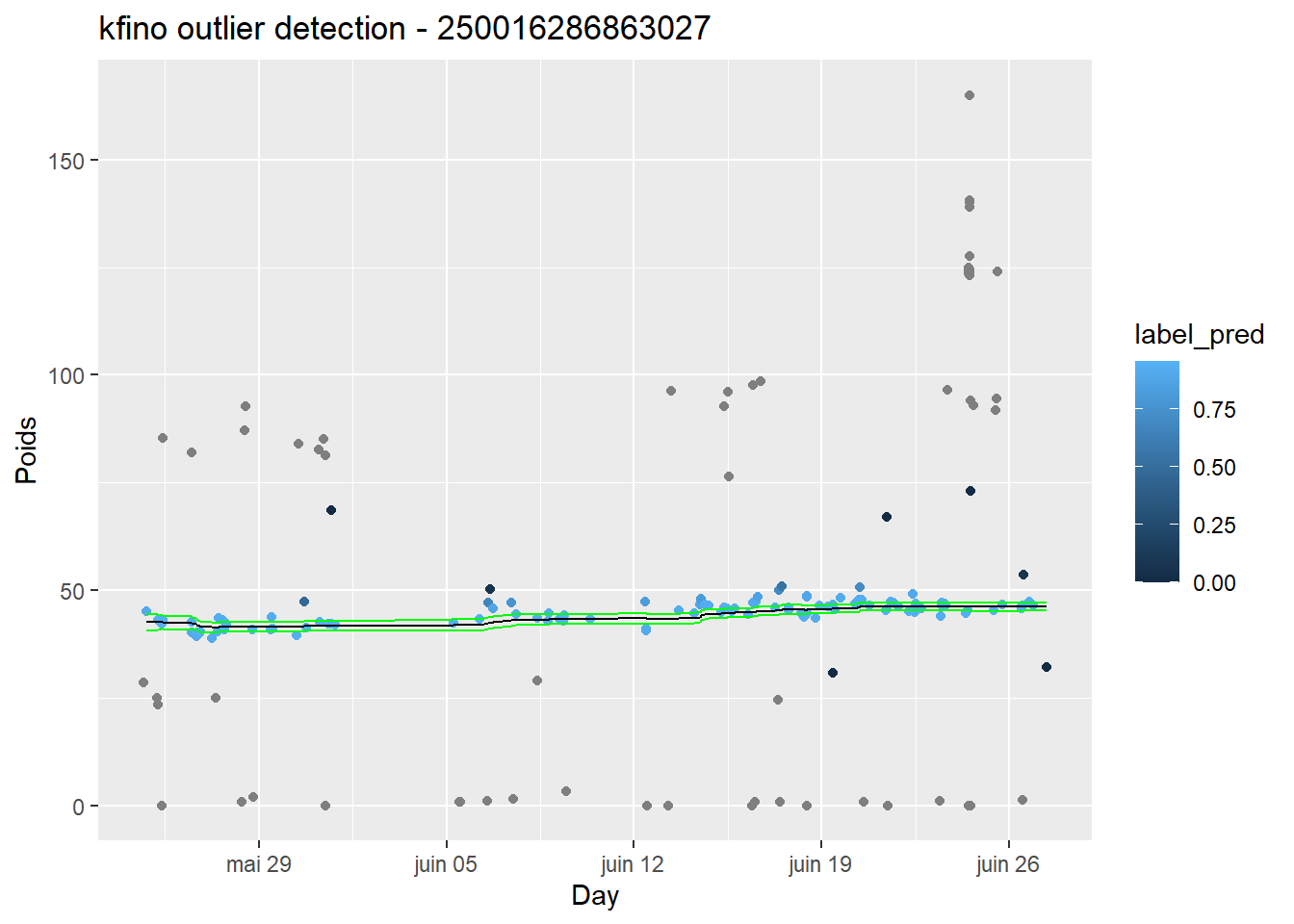``````library(kfino)
library(dplyr)
#>
#> Attachement du package : 'dplyr'
#> Les objets suivants sont masqués depuis 'package:stats':
#>
#>     filter, lag
#> Les objets suivants sont masqués depuis 'package:base':
#>
#>     intersect, setdiff, setequal, union
library(ggplot2)``````

# 1 Objectives

This vignette describes how to use the kfino algorithm on time courses in order to detect impulse noised outliers and predict the parameter of interest.

Kalman filter with impulse noised outliers (kfino) is a robust sequential algorithm allowing to filter data with a large number of outliers. This algorithm is based on simple latent linear Gaussian processes as in the Kalman Filter method and is devoted to detect impulse-noised outliers. These are data points that differ significantly from other observations. `ML` (Maximization Likelihood) and `EM` (Expectation-Maximization algorithm) algorithms were implemented in `kfino`.

The method is described in full details in the following arxiv preprint: https://arxiv.org/abs/2208.00961.

To test the kfino algorithm, we enclosed real data sets into the kfino package. Those data sets were created for the publication describing the mobile and automated walk-over-weighing system:

https://doi.org/10.1016/j.compag.2018.08.022

To test the feasibility of using an automated weighing prototype suitable for a range of contrasting sheep farming systems, the authors automatically recorded the weight of 15 sheep grazing outdoor in spring.

The kfino package has 4 data sets available of automated weighing:

• `spring1`: contains the weighing data of one animal grazing outdoor in spring (203 data points recorded)
• `merinos1`: contains the weighing data of one merinos lamb (397 data points recorded)
• `merinos2`: contains the weighing data of one merinos lamb difficult to model without an appropriate method like kfino (345 data points recorded)
• `lambs`: contains the weighing data of four merinos lambs

# 2 Description of the `spring1` dataset

We start by using the `spring1` data set:

``````data(spring1)

# Dimension of this dataset
dim(spring1)
#>  203   5

#> # A tibble: 6 × 5
#> # Groups:   IDE 
#>   Poids Date                IDE             Day                 dateNum
#>   <dbl> <dttm>              <chr>           <dttm>                <dbl>
#> 1  28.6 2017-05-24 00:00:00 250016286863027 2017-05-24 16:34:00   0.469
#> 2  45   2017-05-24 00:00:00 250016286863027 2017-05-24 19:24:00   0.587
#> 3  25   2017-05-25 00:00:00 250016286863027 2017-05-25 05:25:00   1.00
#> 4  43   2017-05-25 00:00:00 250016286863027 2017-05-25 05:45:00   1.02
#> 5  23.4 2017-05-25 00:00:00 250016286863027 2017-05-25 05:58:00   1.03
#> 6   0   2017-05-25 00:00:00 250016286863027 2017-05-25 09:30:00   1.17``````

The range weight of this animal is between 30 and 75 kg and must be given in `param`, a list of initial parameters to include in the `kfino_fit()` function call.

The user can either perform an outlier detection (and prediction) given initial parameters or on optimized initial parameters (on m0, mm and pp). `param` list is composed of:

• m0 = (optional) the initial weight, NULL if the user wants to optimize it,
• mm = (optional) the target weight, NULL if the user wants to optimize it,
• pp = (optional) the probability to be correctly weighed, NULL if the user wants to optimize it,
• aa = the rate of weight change, default 0.001,
• expertMin = the minimal weight expected by the user,
• expertMax = the maximal weight expected by the user,
• sigma2_m0 = the variance of m0, default 1,
• sigma2_mm = the variance of mm, related to the unit of Tvar, default 0.05,
• sigma2_pp = the variance of pp, related to the unit of Yvar, default 5,
• K = a constant value in the outlier function (trapezium), by default K=5,
• seqp = a vector, sequence of pp probability to be correctly weighted. default seq(0.5,0.7,0.1)

# 3 Kfino algorithm on the `spring1` dataset

## 3.1 Parameters (m0, mm and pp) not optimized

If the user chooses to not optimize the initial parameters, all the list must be completed according to expert knowledge of the data set. Here, the user supposes that the initial weight is around 41 and the target one around 45.

``````# --- Without Optimisation on parameters
param2<-list(m0=41,
mm=45,
pp=0.5,
aa=0.001,
expertMin=30,
expertMax=75,
sigma2_m0=1,
sigma2_mm=0.05,
sigma2_pp=5,
K=2,
seqp=seq(0.5,0.7,0.1))

resu2<-kfino_fit(datain=spring1,
Tvar="dateNum",Yvar="Poids",
param=param2,
doOptim=FALSE,
verbose=TRUE)
#>  "-------:"
#>  "No optimization of initial parameters:"
#>  "Used parameters: "
#>  41.0  0.5 45.0``````

resu2 is a list of 3 elements:

• detectOutlier: The whole input data set with the detected outliers flagged and the prediction of the analyzed variable. the following columns are joined to the columns present in the input data set:

• prediction: the parameter of interest - Yvar - predicted
• label_pred: the probability of the value being well predicted
• lwr: lower bound of the confidence interval of the predicted value
• upr: upper bound of the confidence interval of the predicted value
• flag: flag of the value (OK value, KO value (outlier), OOR value (out of range values defined by the user in `kfino_fit`)
• PredictionOK: A subset of `detectOutlier` data set with the predictions of the analyzed variable on possible values (OK and KO values)

• kfino.results: kfino results (a list of vectors, prediction, probability to be an outlier , likelihood, confidence interval of the prediction and the flag of the data) on input parameters that were optimized if the user chooses this option

``````# structure of detectOutlier data set
str(resu2\$detectOutlier)
#> 'data.frame':    203 obs. of  11 variables:
#>  \$ Poids     : num  28.6 45 25 43 23.4 0 42.2 43 85.4 40.1 ...
#>  \$ Date      : POSIXct, format: "2017-05-24" "2017-05-24" ...
#>  \$ IDE       : chr  "250016286863027" "250016286863027" "250016286863027" "250016286863027" ...
#>  \$ Day       : POSIXct, format: "2017-05-24 16:34:00" "2017-05-24 19:24:00" ...
#>  \$ dateNum   : num  0.469 0.587 1.004 1.018 1.027 ...
#>  \$ rowNum    : int  1 2 3 4 5 6 7 8 9 10 ...
#>  \$ prediction: num  NA 41.5 NA 41.7 NA ...
#>  \$ label_pred: num  NA 0.68 NA 0.88 NA NA 0.9 0.88 NA 0.87 ...
#>  \$ lwr       : num  NA 39.5 NA 39.9 NA ...
#>  \$ upr       : num  NA 43.4 NA 43.5 NA ...
#>  \$ flag      : chr  "OOR" "OK" "OOR" "OK" ...

# head of PredictionOK data set
#>   rowNum prediction label_pred      lwr      upr flag
#> 1      2   41.45659       0.68 39.49659 43.41659   OK
#> 2      4   41.68643       0.88 39.88262 43.49024   OK
#> 3      7   41.75829       0.90 40.07535 43.44123   OK
#> 4      8   41.90155       0.88 40.32265 43.48044   OK
#> 5     10   41.71243       0.87 40.14772 43.27714   OK
#> 6     11   41.81293       0.89 40.33186 43.29400   OK

# structure of kfino.results list
str(resu2\$kfino.results)
#> List of 6
#>  \$ prediction: num [1:121] 41.5 41.7 41.8 41.9 41.7 ...
#>  \$ label     : num [1:121] 0.685 0.875 0.895 0.884 0.874 ...
#>  \$ likelihood: num [1, 1] 1.25e-150
#>  \$ lwr       : num [1:121] 39.5 39.9 40.1 40.3 40.1 ...
#>  \$ upr       : num [1:121] 43.4 43.5 43.4 43.5 43.3 ...
#>  \$ flag      : chr [1:121] "OK" "OK" "OK" "OK" ...``````

Using the `kfino_plot()`function allows the user to visualize the results:

``````# flags are qualitative
kfino_plot(resuin=resu2,typeG="quali",
Tvar="Day",Yvar="Poids",Ident="IDE")````````````
# flags are quantitative
kfino_plot(resuin=resu2,typeG="quanti",
Tvar="Day",Yvar="Poids",Ident="IDE")``````## 3.2 Parameters (m0, mm and pp) optimized

The user can use either (Maximization Likelihood) `ML` or (Expectation-Maximization algorithm) `EM` method.

If the user chooses to optimize the initial parameters, m0, mm and pp must be set to NULL.

``````# --- With Optimisation on parameters
param1<-list(m0=NULL,
mm=NULL,
pp=NULL,
aa=0.001,
expertMin=30,
expertMax=75,
sigma2_m0=1,
sigma2_mm=0.05,
sigma2_pp=5,
K=2,
seqp=seq(0.5,0.7,0.1))``````

### 3.2.1 Maximized Likelihood (ML) method

``````
resu1<-kfino_fit(datain=spring1,
Tvar="dateNum",Yvar="Poids",
param=param1,
doOptim=TRUE,
method="ML",
verbose=TRUE)
#>  "-------:"
#>  "Optimization of initial parameters with ML method - result:"
#>  "no sub-sampling performed:"
#> range m0:  40.1 43
#> initial m0opt:  41.3
#> initial mmopt:  46.3
#>  "Optimized parameters: "
#> Optimized m0:  42.1
#> Optimized mm:  61.1
#> Optimized pp:  0.7
#>  "-------:"

# flags are qualitative
kfino_plot(resuin=resu1,typeG="quali",
Tvar="Day",Yvar="Poids",Ident="IDE")````````````
# flags are quantitative
kfino_plot(resuin=resu1,typeG="quanti",
Tvar="Day",Yvar="Poids",Ident="IDE")``````Prediction of the weight on the cleaned dataset:

``````kfino_plot(resuin=resu1,typeG="prediction",
Tvar="Day",Yvar="Poids",Ident="IDE")``````